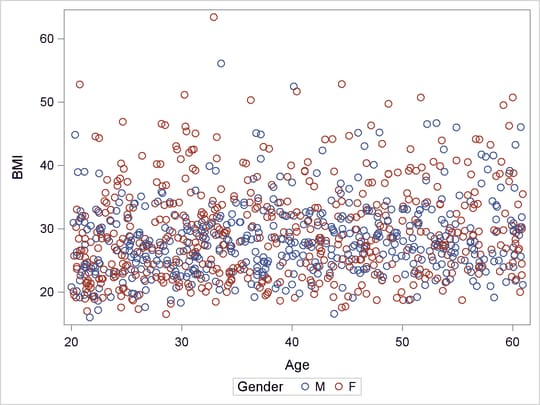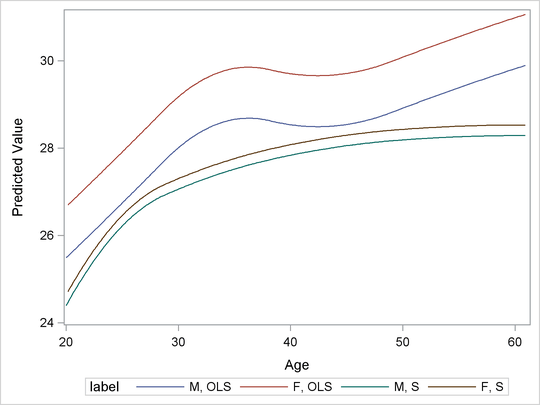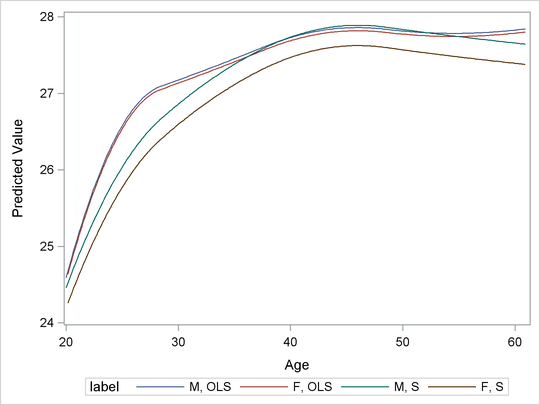### Example 80.4 Constructed Effects

The algorithms of PROC ROBUSTREG assume that a response variable is linearly dependent on the regressors. However, in practice, a response often depends on some factors in a nonlinear manner. This example demonstrates how a nonlinear response-factor relationship can be modeled by using constructed effects. (See the section EFFECT Statement of Chapter 19: Shared Concepts and Topics, for details.)

The following data set contains 526 female observations and 474 male observations sampled from 2003 National Health and Nutrition Examination Survey (NHANES). Each observation is composed of three values: `BMI` (body mass index), `Age`, and `Gender`, measured for subjects whose ages are between 20 and 60.

```data BMI;
input BMI Age Gender \$ @@;
datalines;
46.16 30.33 F 20.67 31.83 F 30.98 51.33 F 30.71 31.42 F
29.81 30.50 M 19.94 25.08 F 29.97 41.67 F 24.48 26.92 F
34.34 51.25 F 20.24 53.67 F 27.72 60.25 F 32.85 41.67 M
22.75 47.50 F 32.78 22.42 F 43.07 29.50 F 38.34 58.50 F
40.03 39.92 F 21.78 56.42 M 28.77 39.83 F 28.77 28.75 F
29.73 54.25 M 33.75 35.67 M 28.48 35.83 M 22.12 29.58 F

... more lines ...

26.98 42.50 F 29.44 39.75 M 25.60 52.67 F 19.30 22.00 F
26.53 27.92 F 23.77 29.00 F 29.86 60.58 M 25.41 44.08 M
26.53 24.83 M 33.33 42.08 F 30.52 32.50 F 31.89 38.17 F
32.20 35.92 F 21.73 26.67 M 32.10 39.33 M 25.13 51.75 M
;
```

The goal of this analysis is to evaluate whether the `BMI` by `Age` curves are different between women and men at a 5% significance level. In order to provide sufficient flexibility to model the effect of `Age` on `BMI`, you can use regression splines that you define with an EFFECT statement. In this example, a regression spline of degree 2 with three knots is used for variable `Age`. The knots are placed at the 25, 50, and 75 percentiles of `Age`. This analysis assume that there is no interaction between `Gender` and `Age`, so that the `BMI` by `Age` curves for women and men are the same up to a constant. The following statements produce the `BMI` by `Age` scatter plot shown in Output 80.4.1:

```proc sort data=bmi;
by age;
run;

ods graphics on;
proc sgplot data=bmi;
scatter x=age y=bmi/group=gender;
run;
```

Output 80.4.1: Scatter Plot for BMI DataThe observations with large `BMI` values (for example, `BMI` > 40) are outliers that can substantially influence an ordinary least squares (OLS) analysis. Output 80.4.1 shows that the distributions of `BMI` conditional on `Age` are skewed toward the side of large `BMI`, and there are more observations with large `BMI` values (outliers) in the female group. Hence you can expect a significant `Gender` difference in the `BMI` by `Age` OLS regression analysis. This expectation is confirmed by the OLS `Gender` p-value = 0.0059 in Output 80.4.2, which is produced by the following statements:

```proc glmselect data=bmi;
class gender;
effect Age_Sp=spl(age/degree=2 knotmethod=percentiles(3));
model bmi = gender age_sp /selection=none showpvalues;
output out=out_ols P=pred R=res;
run;
```

Output 80.4.2: OLS Estimates

The GLMSELECT Procedure
Least Squares Model (No Selection)

Parameter Estimates
Parameter DF Estimate Standard Error t Value Pr > |t|
Intercept 1 29.890089 1.022825 29.22 <.0001
Gender F 1 1.167332 0.422565 2.76 0.0058
Gender M 0 0 . . .
Age_Sp 1 1 -4.404487 1.473761 -2.99 0.0029
Age_Sp 2 1 -3.329537 1.374096 -2.42 0.0156
Age_Sp 3 1 -0.966875 1.314964 -0.74 0.4623
Age_Sp 4 1 -1.611621 1.123854 -1.43 0.1519
Age_Sp 5 1 -0.484787 1.701281 -0.28 0.7757
Age_Sp 6 0 0 . . .

A robust regression method can reduce the outlier influence by automatically assigning smaller or even zero weights to outliers. For the BMI data, a robust regression method is likely to set less weight on observations with large BMI, so more female observations would receive smaller weights than male observations. The following statements invoke PROC ROBUSTREG with the `BMI` data set:

```proc robustreg data=bmi method=s seed=100;
class gender;
effect Age_Sp=spl(age/degree=2 knotmethod=percentiles(3));
model bmi = gender age_sp;
output out=out_s P=pred R=res;
run;
```

Output 80.4.3 shows the parameter estimates and the diagnostics summary produced by PROC ROBUSTREG with the S method. In contrast to OLS, the robust p-value=0.5573 of the `Gender` coefficient indicates that the `Gender` effect is not significant. The outlier diagnostics based on the S estimates find 19 outliers that are assigned lower weights by the S method than by the OLS method.

Output 80.4.3: S Estimates and S Diagnostics Summary

The ROBUSTREG Procedure

Parameter Estimates
Parameter   DF Estimate Standard Error 95% Confidence Limits Chi-Square Pr > ChiSq
Intercept   1 28.2858 1.0081 26.3100 30.2616 787.33 <.0001
Gender F 1 0.2409 0.4114 -0.5654 1.0473 0.34 0.5581
Gender M 0 0.0000 . . . . .
Age_Sp 1 1 -3.8956 1.4376 -6.7133 -1.0779 7.34 0.0067
Age_Sp 2 1 -1.8692 1.3430 -4.5014 0.7630 1.94 0.1640
Age_Sp 3 1 -0.8336 1.2877 -3.3574 1.6903 0.42 0.5174
Age_Sp 4 1 -0.2329 1.1055 -2.3997 1.9338 0.04 0.8331
Age_Sp 5 1 0.0055 1.6632 -3.2543 3.2652 0.00 0.9974
Age_Sp 6 0 0.0000 . . . . .
Scale   0 6.1715

Diagnostics Summary
Observation Type Proportion Cutoff
Outlier 0.0190 3.0000

To further compare the OLS and S outputs, the following statements plot the `BMI` predictions in variable `Age` for both methods in the same graph, which is shown in Output 80.4.4:

```data out2_s;
set out_s;
if gender="F" then label="F, S  ";
if gender="M" then label="M, S  ";
run;

data out2_ols;
merge bmi out_ols;
if gender='F' then label='F, OLS';
if gender='M' then label='M, OLS';
keep pred bmi gender age label;
run;

data out2;
set out2_ols out2_s;
run;

proc sgplot data=out2;
series x=age y=pred/group=label;
run;
```

Output 80.4.4: OLS and S PredictionsYou can observe the following differences between the OLS and S predictions:

• The OLS prediction is larger

• The OLS curves have a local maximum near `Age` = 35

Then, a question remains: is the significance of the `Gender` effect for the OLS regression due solely to the outlying observations? To tentatively answer this question, the following statements drop the observations with the top 10% of `BMI` values from the original data set and reapply OLS and S methods on the reduced data set:

```data three;
set bmi;
where bmi<38.315;
run;

proc robustreg data=three method=s seed=100;
class gender;
effect Age_Sp=spl(age/degree=2 knotmethod=percentiles(3));
model bmi = gender age_sp;
output out=out_s P=pred R=res;
run;
```
```data out2_s;
set out_s;
if gender="F" then label="F, S  ";
if gender="M" then label="M, S  ";
run;

proc glmselect data=three outdesign=four;
class gender;
effect Age_Sp=spl(age/degree=2 knotmethod=percentiles(3));
model bmi = gender age_sp /selection=none showpvalues;
output out=out_ols P=pred R=res;
run;
```
```data out2_ols;
merge three out_ols;
if gender='F' then label='F, OLS';
if gender='M' then label='M, OLS';
keep pred bmi gender age label;
run;

data out2;
set out2_ols out2_s;
run;

proc sgplot data=out2;
series x=age y=pred/group=label;
run;
ods graphics off;
```

Output 80.4.5: S Estimates

The ROBUSTREG Procedure

Parameter Estimates
Parameter   DF Estimate Standard Error 95% Confidence Limits Chi-Square Pr > ChiSq
Intercept   1 27.6427 0.9741 25.7334 29.5520 805.23 <.0001
Gender F 1 -0.2650 0.4023 -1.0535 0.5234 0.43 0.5100
Gender M 0 0.0000 . . . . .
Age_Sp 1 1 -3.1859 1.4032 -5.9361 -0.4356 5.15 0.0232
Age_Sp 2 1 -1.5354 1.3051 -4.0934 1.0226 1.38 0.2394
Age_Sp 3 1 -0.3776 1.2499 -2.8273 2.0721 0.09 0.7626
Age_Sp 4 1 0.3299 1.0668 -1.7610 2.4208 0.10 0.7572
Age_Sp 5 1 0.0949 1.6221 -3.0845 3.2742 0.00 0.9534
Age_Sp 6 0 0.0000 . . . . .
Scale   0 4.9440

Output 80.4.6: OLS Estimates

The GLMSELECT Procedure
Least Squares Model (No Selection)

Parameter Estimates
Parameter DF Estimate Standard Error t Value Pr > |t|
Intercept 1 27.841568 0.780817 35.66 <.0001
Gender F 1 -0.040924 0.317749 -0.13 0.8976
Gender M 0 0 . . .
Age_Sp 1 1 -3.253964 1.121292 -2.90 0.0038
Age_Sp 2 1 -0.975273 1.034172 -0.94 0.3459
Age_Sp 3 1 -0.508979 0.999609 -0.51 0.6108
Age_Sp 4 1 0.089393 0.852774 0.10 0.9165
Age_Sp 5 1 -0.113706 1.298157 -0.09 0.9302
Age_Sp 6 0 0 . . .

Output 80.4.7: OLS and S Predictions on the Reduced Data SetIn the reduced data set, 71 female observations and 29 male observations are dropped. Output 80.4.5 and Output 80.4.6 respectively show the refitted S and OLS parameter estimates, and Output 80.4.7 displays the fitted curves on the reduced data set. You can see that `Gender` is no longer significant for the OLS model, and the OLS turning pattern has also disappeared, but the new S curves do not change much from the previous ones. The OLS `BMI` by `Age` curves in Output 80.4.7 are closer to the S curves than to the OLS curves in Output 80.4.4. This suggests that indeed the difference between the OLS and S estimate results are due solely to the influence of the outlying observations.